# Externalizing Some Simple Topos Statements

### 13 Dec 2022

Hey all! It’s been a minute. I’ve been super busy with the UC strike and honestly I haven’t done math in any serious capacity for almost the past month. It’s been a lot of hard work trying to get fair contracts out of the UC, but I had a lot of travel plans this December to see my friends, so I’ve taken a step back from the picket line until January. Right now I’m in Chicago, so here’s an obligatory bean pic: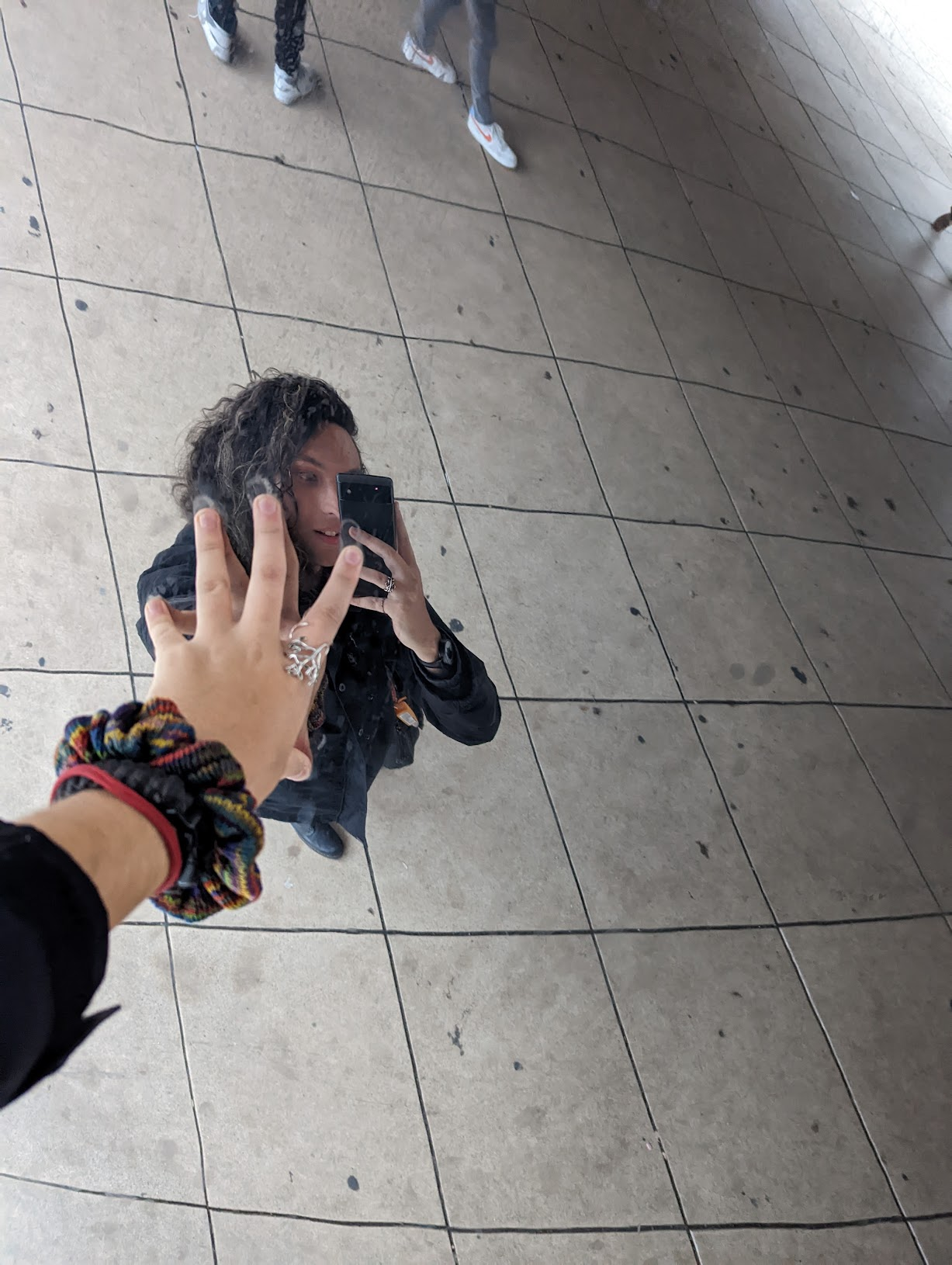This means I have time to do math again, and it’s been really good for me to get back into it! I’ve started working with Peter Samuelson on some really cool work involving skein algebras, and I can’t wait to chat about it once I spend more time with it.

I also spent some time trying to understand manifolds in a topos of $G$-sets. I gave a (fairly informal) talk in Dave Weisbart’s seminar, where I tried to pitch him topos theory as a method of studying $G$-invariant constructions1, and I thought I remembered a result that we can get our hands on a global quotient orbifold $M // G$ by working with a manifold internal to $G$-set… I couldn’t find a reference for this, so I set about trying to prove it myself, and (to nobody’s surprise) it’s false, haha.

So my last couple weeks, when I had the energy to do math after picketing, looked eerily like Julia Robinson’s famous description of her week: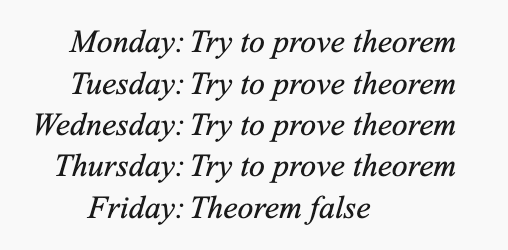I learned a ton while doing this, though, and I wanted to share some insights with everyone. It’s really hard to find examples of people taking statements in the internal logic of a topos and externalizing them to get “classical” statements, so I had to work out a bunch of small examples myself.

Let’s go over a few together, and hopefully make things easier for the next round of topos theorists looking to do this ^_^

$\lvert X \rvert = 3$

Let’s start small. What does it mean to say that $$\lvert X \rvert = 3$$? This is really an abbreviation saying “there’s a bijection between $X$ and $3$”, which we expand out to

$\exists \alpha : 3^X . \exists \beta : X^3 . \alpha \beta = \text{id}_3 \land \beta \alpha = \text{id}_X$

Here, of course, we’re writing $A^B$ for the exponential object of the topos.

So we’re saying that $$\mathcal{E} \models \lvert X \rvert = 3$$. If we switch over to the forcing language, we’re saying that $$1 \Vdash \lvert X \rvert = 3$$, and from here we can follow the instructions in section VI.6 of Mac Lane and Moerdijk’s Sheaves in Geometry and Logic. In fact, since we’ll be working exclusively with grothendieck topoi in this post, we can work with the slightly simpler sheaf semantics outlined in section VI.7.

First, let’s look at the case $\mathcal{E} = G\text{-}\mathsf{Set}$.

$1 \Vdash \exists \alpha : 3^X . \exists \beta : X^3 . \alpha \beta = \text{id}_3 \land \beta \alpha = \text{id}_X$

Next we cash out our existential quantifiers for honest (generalized) elements $f$ in $3^X$ and $g$ in $X^3$. The “local” nature of existential quantification, though, means that our generalized elements no longer have domain $1$ (which would make them global elements). Instead, they have domain $V$ for some epi $V \twoheadrightarrow 1$.

Of course, in a topos of $G$-sets, every nonempty object admits a unique epi to $1$, and every nonempty object is a disjoint union of $G$-orbits. So it’s not hard to see we can restrict attention to the connected epis (transitive $G$-sets). But we also know that truth is local. So we can pull back some transitive $G$-set along a further epimorphism to assume that our connected object is actually $G$ itself!

So we end up with elements $\alpha : G \to 3^X$ and $\beta : G \to X^3$ so that

$G \Vdash \alpha \beta = \text{id}_3 \land \beta \alpha = \text{id}_X$

But what does this mean? Well, in $G$-set, the exponential $A^B$ is the set of all functions ${\varphi : B \to A}$ equipped with the conjugation action:

$(g \cdot_{A^B} \varphi)(x) = g \cdot_A \varphi (g^{-1} \cdot_B x)$

Notice that the global elements of $A^B$ are the maps $1 \to A^B$, which are thus the fixed points of $A^B$. But to say $\varphi = g \cdot \varphi$ for all $g$ is to say that $\varphi$ is $G$-equivariant. However generalized elements give us access to other maps. In particular:

Exercise: Show that the $G$-elements of $A^B$ (that is, the maps $G \to A^B$) are in natural bijection with ordinary functions $B \to A$ that ignore the $G$-structure entirely.

Hint: Look at what happens to the identity element of the group

So then we have two ordinary functions $\alpha : X \to 3$ and $\beta : 3 \to X$ which are mutually inverse. This means exactly that the underlying set of $X$ has $3$ elements.

This brings us to an important observation about $G$-sets:

Something is “locally” true in $G\text{-}\mathsf{Set}$ exactly when it’s true for the underlying sets. This is basically because each $G$-set $X$ is covered by $G \times X$, but this is isomorphic to $G \times UX$ where $UX$ (the underlying set of $X$) is equipped with the trivial $G$-action!

So if we want a statement in $G\text{-}\mathsf{Set}$ to externalize to something $G$-equivariant, we’ll want to avoid existential quantifiers and disjunctions (since these are only true up to a cover)2.

Can you find a formula $\psi(X)$ (which will use $G$ as a parameter) so that $G\text{-}\mathsf{Set} \models \psi(X)$ if and only if $X$ has finitely many $G$-orbits3?Say that instead of $G$-sets, we work inside a topos $\mathcal{E} = \mathsf{Sh}(S)$ for some topological space $S$4. What does it mean if $$\mathcal{E} \models \lvert X \rvert = 3$$?

Well again, we see that

$1 \Vdash \exists \alpha : 3^X . \exists \beta : X^3 . \alpha \beta = \text{id}_3 \land \beta \alpha = \text{id}_X$

and we cash out our existential quantifiers for local witnesses. That is, there’s an open cover ${ U_i }$ of $S$ with elements $\alpha_i : U_i \to 3^X$ and $\beta_i : U_i \to X^3$ so that, for each $U_i$,

$U_i \Vdash \alpha_i \beta_i = \text{id}_3 \land \beta_i \alpha_i = \text{id}_X$

Now (by yoneda), an element $U_i \to A^B$ is “just” a function $B \to A$ defined over $U_i$. So altogether we see that $\lvert X \rvert = 3$ if and only if there’s an open cover $$\{U_i\}$$ of $S$ so that $$X \! \upharpoonright_{U_i} \cong 3 \! \upharpoonright_{U_i}$$ for each $i$.

This means $X$ is locally isomorphic to $3$, the disjoint union of three copies of $S$. It’s not hard to see that this means $X$ is a 3-sheeted covering space of $S$.

Notice that these local isomorphisms do not have to glue into a global isomorphism! The simplest thing to do is to give a picture. If $S = S^1$ is a circle, and $X$ is a triple cover as shown below: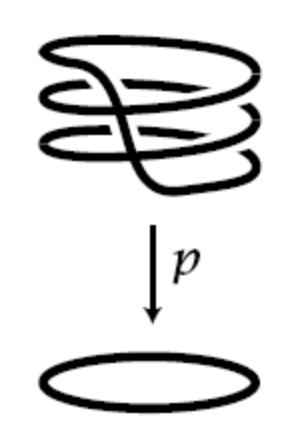then $X$ is locally isomorphic to the trivial triple cover $3$: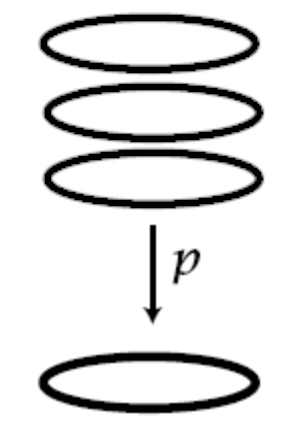despite the fact that they aren’t globally isomorphic!

From the perspective of the internal logic, the point is that the isomorphisms over each $U_i$ need not be compatible in the sense that $$\alpha_i \! \upharpoonright_{U_i \cap U_j} \neq \alpha_j \! \upharpoonright_{U_i \cap U_j}$$.

If you’ve never done it before, it’s worth going through this in detail!

Cover $S^1$ by open sets (so that each intersection is connected, for simplicity) and show that the two triple covers are isomorphic on each open set, but these isomorphisms aren’t compatible on intersections.

Note, as an aside, that we’ve been working with an explicit choice of finite cardinality: $\lvert X \rvert = 3$. Say instead we wanted the weaker “$\lvert X \rvert \text{ is finite}$”. There’s nothing to worry about in $G\text{-}\mathsf{Set}$ because this topos is boolean, satisfies AC, etc. However for topoi without LEM, there are multiple inequivalent notions of finiteness. See here for a discussion5.

$\forall x,y : X . x = y \lor x \neq y$

This says that $X$ is decidable, which is language coming from a computability theoretic interpretation of our logic that I’ll save for a different post6.

Let’s start with the externalization. If $\mathcal{E} \models \forall x, y : X . x = y \lor x \neq y$, then in the forcing notation we have

$1 \Vdash \forall x, y : X . x = y \lor x \neq y$

which we can cash out (again, see chapter VI in Mac Lane and Moerdijk) for

$X \times X \Vdash \pi_1 = \pi_2 \lor \pi_1 \neq \pi_2$

where $\pi_1$ and $\pi_2$ are the projections $X \times X \to X$.

Now, just like existential quantifiers, disjunctions need only be true locally. So in the case $\mathcal{E} = G\text{-}\mathsf{Set}$ we want to find $G$-sets $V$ and $W$, with maps $p: V \to X^2$ and $q : W \to X^2$ so that

1. $V \Vdash \pi_1 p = \pi_2 p$
2. $W \Vdash \pi_1 q \neq \pi_2 q$
3. $p+q : V + W \to X^2$ is an epi

Of course, this isn’t hard to do! Let’s take $$V = \{ (x,x) \mid x \in X \}$$ (equipped with the diagonal $G$-action) and $$W = X^2 \setminus V$$ (also equipped with the diagonal $G$-action).

If it’s not obvious, prove that $W$ really is a $G$-set.

Then it’s easy to see that by taking $p$ and $q$ to be the relevant inclusion maps we can satisfy our 3 conditions. Thus every $G$-set $X$ is decidable!7

Next, let’s look at $\mathsf{Sh}(S)$.

Again, we’ll take an object $X$ and look at the statement $\forall x,y : X . x = y \lor x \neq y$.

The flow of the computation is hopefully becoming familiar now. For variety, since we’re working in a sheaf topos, let’s do this computation with the sheaf semantics (Maclane and Moerdijk VI.7). This lets us restrict attention to open subsets of $S$, which is sometimes useful.

We start with $1 \Vdash \forall x,y : X . x = y \lor x \neq y$, that is, $S \Vdash \forall x,y : X . x = y \lor x \neq y$.

Now the universal quantifiers represent true statements for any local section. That is, for each $U$ open in $S$, and for each generalized element $x,y : U \to X$ (that is, by yoneda, local sections $x,y \in X(U)$ of the sheaf $X$ over $U$)

$U \Vdash x = y \lor x \neq y$

Then since disjunctions need only be true locally, this is true exactly when we can over $U$ by opens $V_i$ so that, on each $V_i$, either

$V_i \Vdash x = y$

or

$V_i \Vdash x \neq y$

That is, on each $V_i$ in the cover, either the sections $x \upharpoonright_{V_i}$ and $y \upharpoonright_{V_i}$ are everywhere equal or they’re nowhere equal.

So an object $X$ in $\mathsf{Sh}(S)$ is decidable exactly when, for any connected open $U \subseteq S$, any two local sections of $X$ are either everywhere equal or nowhere equal8.

For a super concrete example, let’s look at $S = [-1,1]$ and $X$ the sheaf of continuous functions on $S$. Then if we look at $x = s$ and $y = \lvert s \rvert$, we can ask about the truth values $x=y$ and $x \neq y$.

Here $x=y$ is the largest open subset of $S = [-1,1]$ on which $s = \lvert s \rvert$. This is, of course, $(0,1]$. Similarly, $x \neq y$ is the largest open subset on which $s \neq \lvert s \rvert$, but her friends call her $[-1,0)$.

Notice the truth values are open subsets of $S$. We’re keeping track of where something is true, which is a finer (and more useful!) tool than just a simple boolean true/false.

But of course, this means that $x=y \lor x \neq y$ is the union $$(0,1] \cup [-1,0) = [-1,1] \setminus \{0\}$$. Which is not the top element of the lattice of opens, and thus is not “true”! Even though $0 = \lvert 0 \rvert$, this truth is not local – No matter how slightly we wiggle $0$, this truth value can change, and this instability is exactly what keeps $0$ from being in the set $$[-1,0) \cup (0,1] = [ \! [ s = |s| \lor s \neq |s| ] \! ]$$

Let’s give a bonus example as well, since this is a fairly subtle concept.

We’ll work in the arrow topos $\mathsf{Set}^{\to}$ whose objects are triples $(a,f,b)$ where $f : a \to b$ in $\mathsf{Set}$. An arrow $(\varphi_0, \varphi_1) : (a,f,b) \to (c,g,d)$ is a pair of arrows in $\mathsf{Set}$ making the obvious square commute: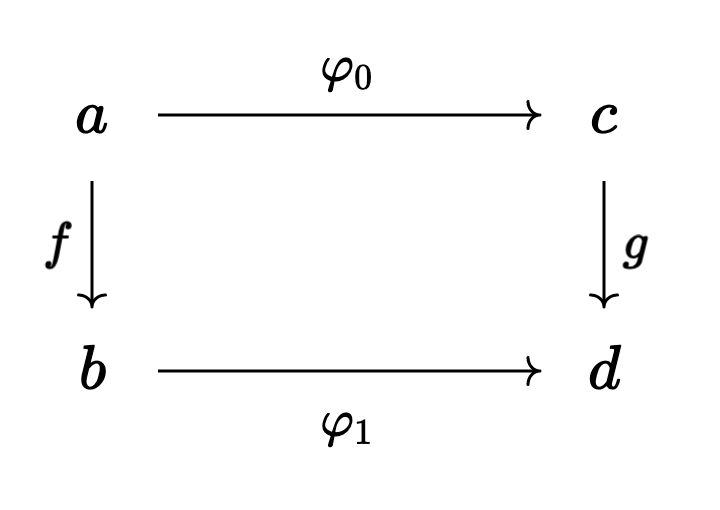I’ll leave much of this example as a fun exercise, since it’s important to get practice working these things out for yourself! If you want to check your work, though, I’ll leave brief solutions in a fold!

First, can you compute the object of truth values $\Omega$?

solution

This is worked out in detail in chapter I of Mac Lane and Moerdijk (pages 35 and 36 of my edition) but briefly, we get

$\Omega = \{ \top, \bot', \bot \} \overset{\sigma}{\to} \{ \top, \bot \}$

with $\sigma(\top) = \top$, $\sigma(\bot’) = \top$, $\sigma(\bot) = \bot$.

Remember that a subobject of $X_0 \overset{f}{\to} X_1$ is a pair of subsets $A_0 \subseteq X_0$, $A_1 \subseteq X_1$ so that $f[A_0] \subseteq A_1$. This is exactly a restriction of $f$!

Then any $x_1 \in X_1$ is either in $A_1$ or it isn’t. That’s why the target of $\sigma$ is $$\{ \top, \bot \}$$. But an $x_0 \in X_0$ has more options. Either

• $x_0 \in A_0$ and, necessarily, $f x_0 \in A_1$
• $x_0 \not \in A_0$ but $f x_0 \in A_1$ anyways
• $x_0 \not \in A_0$ and $f x_0 \not \in A_1$

these correspond to the truth values $\top$, $\bot’$, and $\bot$ in the domain of $\sigma$.

Next, can you figure out what it means for an object $a \overset{f}{\to} b$ to be decidable?

solution

Let’s compute.

We quickly get to $f^2 \Vdash x=y \lor x \neq y$, where (as usual) $x$ and $y$ are the projection maps from $f^2 \to f$.

Another, smaller, computation shows that $f^2 : a^2 \to b^2$ is just the map sending $(x,y) \mapsto (fx,fy)$. Moreover, an epi

$(\tilde{a} \overset{\tilde{f}}{\to} \tilde{b}) \twoheadrightarrow (a \overset{f}{\to} b)$

is a pair of surjections $\tilde{a} \to a$ and $\tilde{b} \to b$ making the square commute.

With this in mind, when we unwind the disjunction to a pair of maps $V \to f^2$ and $W \to f^2$ so that

• $V \Vdash x=y$
• $W \Vdash x \neq y$
• $V+W \to f^2$ is epi

it’s enough to look at the images of the maps $V \to f^2$ and $W \to f^2$. That is, it’s enough to look at $V$ and $W$ subobjects of $f^2$ and ask that the union $V \cup W$ is all of $f^2$.

To have our best chance at covering $f^2$, we should take $V$ and $W$ to be as big as possible under the restrictions that $V \Vdash x=y$ and $W \Vdash x \neq y$.

Unwinding $V \Vdash x=y$, the best we can do is to take $V$ to be the diagonal $$\{ (x,y) \in a^2 \mid x=y \} \overset{f^2}{\to} \{ (x,y) \in b^2 \mid x=y \}$$.

Then unwinding $W \Vdash x \neq y$, the best we can do is $$\{ (x,y) \in a^2 \mid x \neq y \land fx \neq fy \} \overset{f^2}{\to} \{ (x,y) \in b^2 \mid x \neq y \}$$.

Notice we have to restrict the domain to those elements who stay separated after we apply $f^2$! After all, if $x \neq y$ but $fx = fy$, then we wouldn’t land in the right codomain9.

So if we want the union of $V$ and $W$ to be all of $f$, we need to know that each pair $(x,y)$ with $x \neq y$ gets sent to a pair with $fx \neq fy$, and this happens exactly when $f$ is a monomorphism!

So the decidable objects of $\mathsf{Set}^\to$ are the monos.

Hopefully this also makes clear, in a more discrete way than the $\mathsf{Sh}(S)$ example, how decidability can fail! For a super concrete example10, consider the ring object in $\mathsf{Set}^\to$ given by

$\mathbb{Z}[\epsilon] / \epsilon^2 \overset{\epsilon \mapsto 0}{\longrightarrow} \mathbb{Z}$

This ring is not decidable since $\epsilon = 0$ is neither true nor false!

This was a lot of fun, and hopefully you feel more comfortable externalizing some simple statements after reading through these. It’s all about practice practice practice, though, so I encourage everyone to come up with their own easy examples and try externalizing them! I learned a ton while writing this blog post, and that’s on top of everything I learned trying to work out what a manifold inside $G\text{-}\mathsf{Set}$ should be!

I won’t make promises, but I would love to write another post of this flavor sometime soon, where we can talk about something simple like linear algebra or basic analysis inside a topos. Of course, I have lots of ideas, and comparatively little time to write them all, so things will happen when they do ^_^.

Stay warm and stay safe, all! We’ll talk soon 💖

1. In particular, we’re planning to think about $G$-equivariant brownian motion.

2. Something like this is probably true for more general etendues, which (up to a cover) look like $\mathsf{Sh}(X)$. The case of $G$-sets is particularly easy, since locally they look like $\mathsf{Set} = \mathsf{Sh}(\star)$.

3. Try $\exists n : \mathbb{N} . \exists f : X^{G \times n} . \ulcorner f \text{ is surjective} \urcorner$

4. Nothing at all changes if we instead take $S$ to be a locale

5. Also note that the existential quantifier really impacts things. Essentially we asked for $\mathcal{E} \models \exists \alpha : X \cong 3 . \top$. If we had instead asked for an actual (global) element, $\mathcal{E} \models \alpha : X \cong 3$, we would have actual isomorphism instead of mere local isomorphism. This is what the previous nlab link calls “(bishop) finite”, and it’s a stronger condition than what we’ve been working with.

6. Briefly, a property is called decidable if a computer can check if the answer is yes or no.

A property is called semidecidable if a computer can check if the answer is “yes”, but we don’t know how long it’ll take. What’s worse, the code is allowed to loop forever if the answer is “no”! So really we get a “yes” or “maybe”.

Dually, we say a property is co-semidecidable if a computer can answer “no” or “maybe”. If you run it long enough and the answer is “no”, it will always say so. But if the answer is “yes” the code might loop forever.

As a (fairly easy?) exercise, show that something is decidable if and only if it’s both semidecidable and co-semidecidable.

To finish the brief explanation, equality is such an important predicate that we say $X$ is decidable exactly when equality on $X$ is, and this is the definition we ported to topos theory.

7. This should make intuitive sense. A topos of $G$-sets is boolean, and even satisfies AC. So it looks almost exactly like $\mathsf{Set}$! In particular, we can see that every object in $G\text{-}\mathsf{Set}$ is decidable by appeal to LEM, rather than by building the witnesses directly.

Yet another way to see this (which I’m 80% sure is true) is by working in the slice topos. I’m pretty sure that

$X^2 \Vdash \pi_1 = \pi_2 \lor \lnot \pi_1 = \pi_2$

is the same thing as asking for

$\pi_1 = \pi_2 \lor \lnot \pi_1 = \pi_2$

to name $\top$ in the slice topos $\mathcal{E} /_{ X^2}$.

Here the truth values are subobjects of $X^2$ in $\mathcal{E}$, with $\pi_1 = \pi_2$ naming the truth value

$\{ (x,y) \mid x = y \}$

and $\lnot \pi_1 = \pi_2$ naming the truth value

$\text{the biggest sub-G-set disjoint from } \{ (x,y) \mid x = y \} \text{''}$

of course, since the complement of the diagonal is a sub-$G$-set, these two sets union to the whole of $X^2$. That is, the truth value of their disjunction is $\top$, as desired.

Note this is not the case for $M$-sets for a monoid $M$!

If we work with the monoid $\mathbb{N}$, let’s consider the $\mathbb{N}$-set $X = {a,b}$ with $1a = 1b = b$. That is,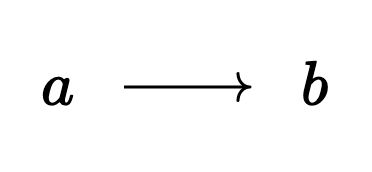(plus a self loop at $b$ that q.uiver won’t draw for me… I’d make it myself but it’s getting late and I don’t feel like it, haha)

Then the product $X^2$ is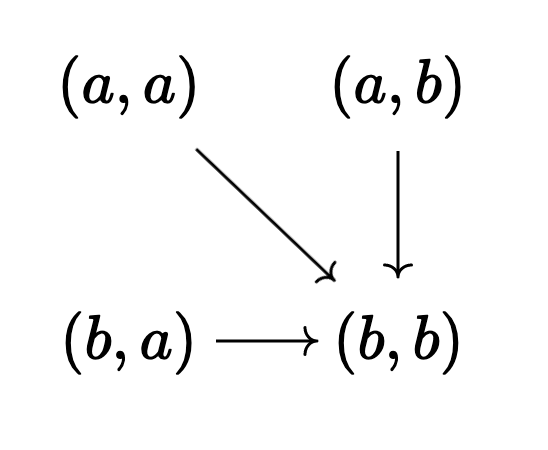(again, with a secret self loop at $(b,b)$).

The diagonal, of course, is a sub-$M$-set: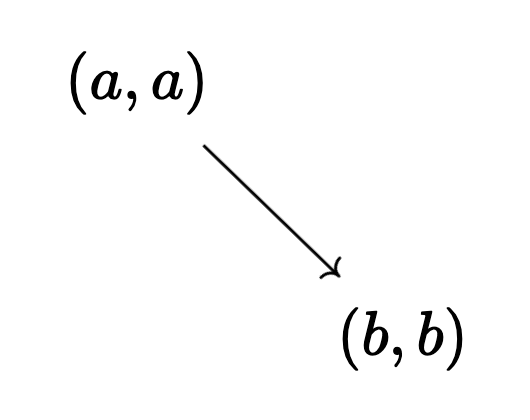But now the off-diagonal is not a sub-$M$-set. It’s not closed under the $M$-action: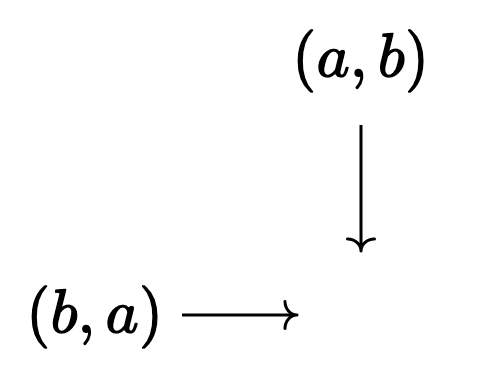So the complement $$\{ (x,y) \mid x \neq y \}$$ will be the largest sub-$M$-set contained inside the off-diagonal. But in this example that’s empty!

In particular, $$\{ (x,y) \mid x=y \} \lor \{ (x,y) \mid x \neq y \}$$, a subobject of $X^2$, and thus a truth value in $$M\text{-}\mathsf{Set} \big /_{\! X^2}$$, is not $X^2$. So $M\text{-}\mathsf{Set}$ thinks $\forall x,y : X . x = y \lor x \neq y$ is not true.

It also thinks it’s not false, for what that’s worth.

As a nice (slightly more challenging) exercise, figure out what the truth value of that statement actually is!

It’s still not fully clear to me what truth values in $M\text{-}\mathsf{Set} /_{X^2}$ should look like… In $M\text{-}\mathsf{Set}$ there are only two global truth values, even though externally we can see that $\Omega$ is the set of all (left) ideals of $M$.

Presumably in $M\text{-}\mathsf{Set} /_{X^2}$ the global truth values are the sub-$M$-sets of $X^2$… But I’m not sure about what exactly $\Omega$ looks like.

If someone wants to work this out, I’d love to hear your thoughts in the comments! This footnote is already getting super long, though, and I have the rest of the post to work on!

8. This is obviously an extremely strict condition! If we think about the sheaf of real-valued continuous functions on $S$, it’s hard to imagine the case that two functions which agree on a point automatically agree everywhere!

9. This is worth some meditation. There’s something model-theoretic happening here, where truth is preserved as we move from the domain to the codomain. But falsity does not need to be preserved (said another way, truth does not need to be reflected from the codomain to the domain).

So true things stay true, but false things can become true later on.

It’s possible for $x$ and $y$ to start different and end the same, but if they start the same they have to stay that way.

Somehow the logic of the topos handles all of this for us, which doesn’t seem so impressive when we only have one arrow, but you can imagine as we increase the complexity of the category $\mathcal{C}$ in the topos $\mathsf{Set}^\mathcal{C}$ that it becomes more annoying to do this bookkeeping by hand.

Regardless, it’s not lost on me that $f$ is decidable in $\mathsf{Set}^\to$ exactly when it’s a monomorphism in $\mathsf{Set}$… This has something to do with the fact that, model theoretically, monos do reflect the truth of atomic questions (like equality). I’m seeing some connection here, but I can’t quite make it precise.

10. taken from Johnstone’s Rings, Fields, and Spectra, which should really be required reading for anyone interested in applications of topos theory!